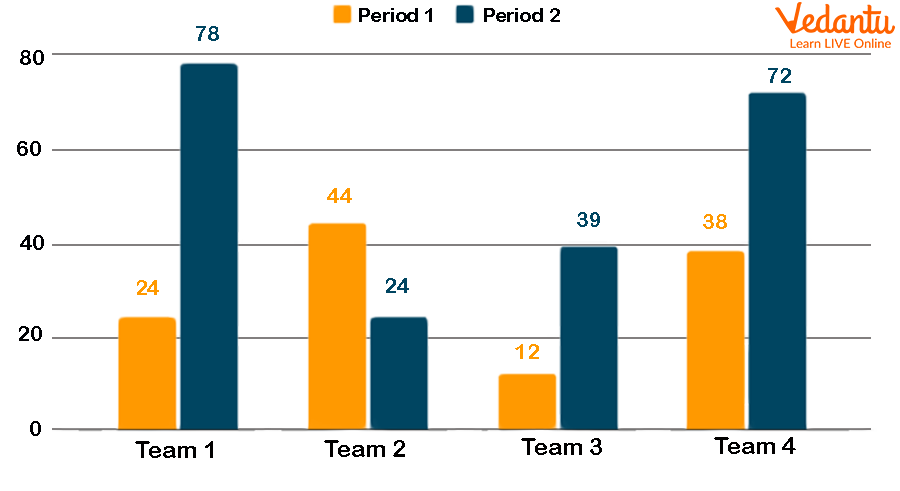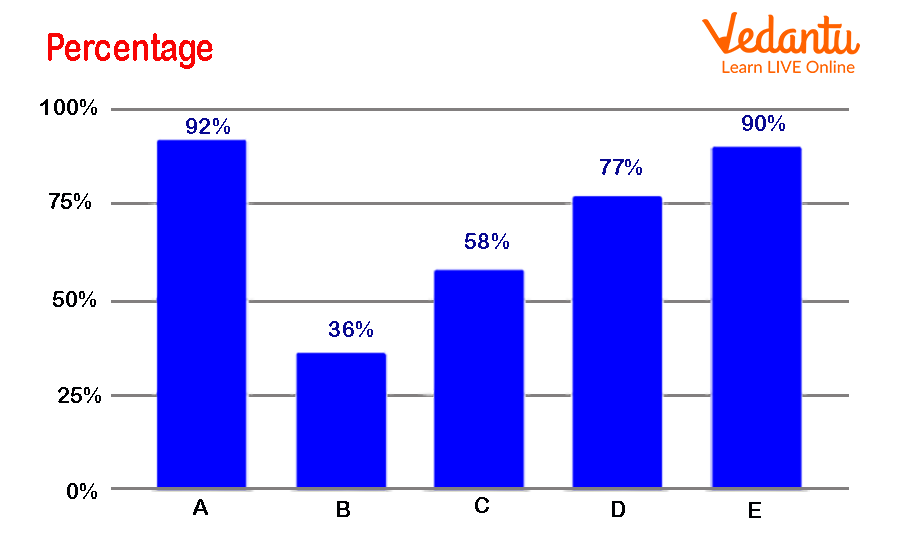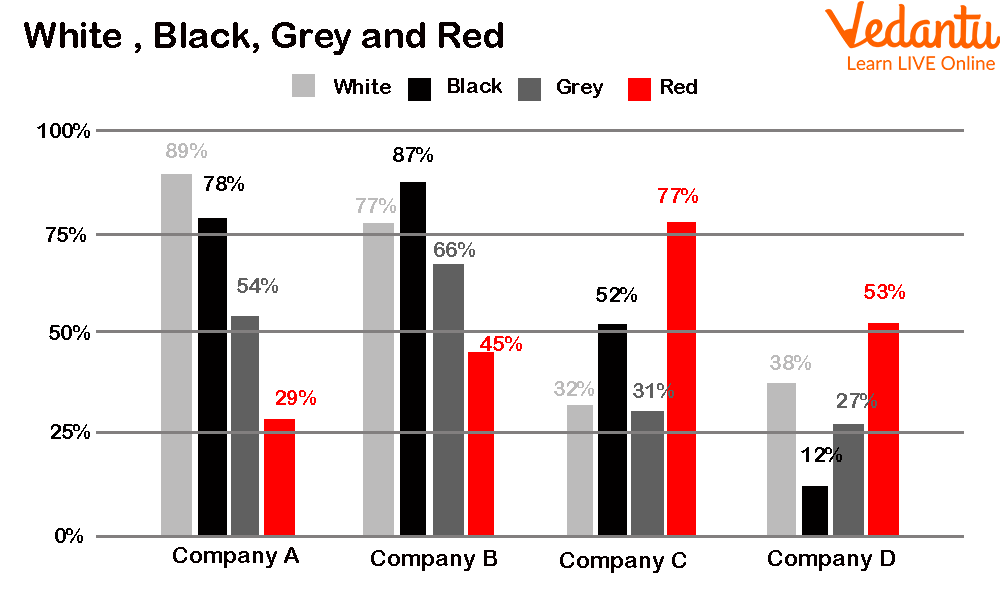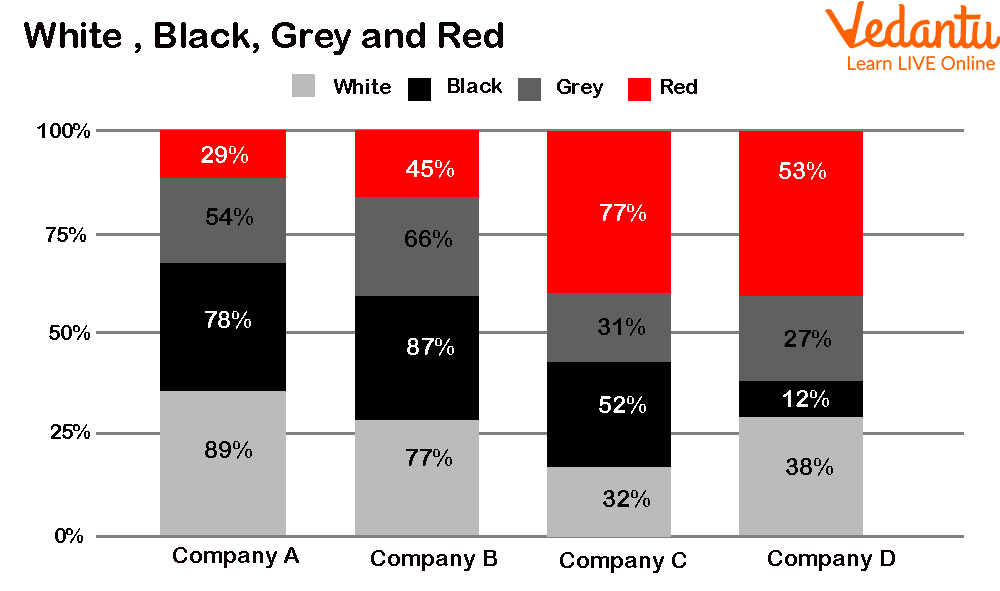Courses
Courses for Kids
Free study material
Free LIVE classes
More

# Vertical Bar Graph and Types of Vertical Bar Graphs## What is a Vertical Bar Graph?

In a vertical bar graph, the bars are plotted vertically along the Y-axis. This type of bar graph is known as a vertical bar graph. These types of graphs are generally drawn for the discrete data types and quantitative comparison between items or observations.

Here, the observation that is going to be compared is represented by the X-axis and the Y-axis represents the parameters on which this comparison is made. The bars of observation originate from the X-axis and are drawn vertically upwards towards the Y-axis.An Example of Representing a Bar Graph

## Types of Vertical Bar Graphs

According to the requirement of the information, there can be different types of vertical bar graphs. Vertical bar graphs are broadly categorised into two types:

1. Simple vertical bar graphs

2. Compound vertical graphs

Now let’s understand these types of graphs.

1. Simple vertical bar graphs

In a simple vertical bar graph, there is only one parameter of comparison for each observation. This means that there will be only one bar for each observation. For example, if 5 students of a class are ranked on the basis of the total percentage of marks, then the single vertical bar graph will look like the figure given below.Example of Simple Vertical Bar Graphs

2. Compound vertical bar graphs

In a compound bar graph, there are at least two or more parameters of comparison for each observation. Hence, there will be two or more bars drawn. In compound vertical bar graphs, there can be confusion between bars of parameters, so different colours are assigned to bars.

Compound vertical bar graphs are further classified into two types:

(i) Multiple vertical bar graphs

(ii) Stacked vertical bar graphs

(i) Multiple vertical bar graphs: In this bar graph, different bars are made for different parameters for each observation. Hence, for each observation, different bars of different colours are drawn to represent the different parameters. For example, a multiple vertical bar graph is shown below for the percentage of sales for 4 different colour cars in a company.Example of Multiple Vertical Bar Graphs

(ii) Stacked vertical bar graphs

In a stacked vertical bar graph, all parameters of one observation are represented in a single bar or the bars are stacked on top of each other. For example, if we represent the same example of 4 different colour car sales in a company, the stacked vertical bar graph will look like the figure given below.Example of Stacked Vertical Bar Graphs

## Conclusion

Graphs and charts are very important; they are helpful in analysing and measuring data in a simple manner. In this article, the vertical bar graph is discussed with its types. Examples of each type of vertical bar graph are also provided.

Last updated date: 24th Sep 2023
Total views: 130.8k
Views today: 4.30k

## FAQs on Vertical Bar Graph and Types of Vertical Bar Graphs

1. What is a vertical and horizontal bar graph?

The bar chart or graph is used to represent some sort of information or data in an easy form where the vertical axis (Y-axis) represents the categories being compared and the horizontal axis (X-axis) represents a value. When the bars of observation originate from the X-axis and are drawn vertically upwards towards the Y-axis, they are called vertical bar graphs, and when the bars of observation originate from the Y-axis and are drawn vertically upwards towards the X-axis, they are called horizontal bar graphs.

2. What is the use of vertical bar graphs?

Vertical bar graphs or charts gave us a visual representation of categorical data. This data is combined together as a group. We often need to analyse data in an easier way. Hence, bar graphs are required. One can easily understand the information and conclude different aspects of data from vertical bar graphs.